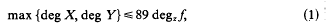Skip to main content Accessibility help
Home

# Norm form equations IV: Rational functions

## Extract

This paper is devoted to the establishment of explicit bounds on the rational function solutions of a general class of equations in several variables. The first general result on Diophantine equations over function fields was discovered in 1930 , as an analogy on the work of Thue on number fields: it was shown that the degrees of polynomial solutions X, Y in k[z] of f(X, Y) = c are bound for each c: here f denotes an irreducible binary form over k[z] of degree at least three, and k is an algebraically closed field of characteristic zero. The Manin-Grauert theorem  extended this conclusion to the rational function solutions X, Y in k(z) of any equation in two variables over k(z), provided that the curve corresponding to the equation has genus two or more: this is the analogue for function fields of the Mordell conjecture for number fields, proved by Faltings in 1983. Parsin  made the Manin-Grauert theorem effective by furnishing explicit bounds on the degrees of X and Y: this approach followed Grauert and Shafarevitch in relying heavily on algebraic geometry. In 1976 a different attack was made by Schmidt  using the theory of algebraic differential equations, first developed by Kolchin and Osgood. This method produced very good bounds for equations such as the Thue equation discussed above: for example, Schmidt provides the boundfor any rational function solution X, Y in k(z) of f(X, Y) = 1, where f denotes a binary form over k(z) of degree in X and Y at least five, and which factorises into distinct linear factors. Here deg zf is the degree of f in z. In 1983  the power of Diophantine approximation provided another approach to attack Dlophantine equations.over function fields. By first establishing an inequality on solutions of the unit equation in two variables, it was shown in [3, p. 122] that the bound (1) could be improved to 36 degz f, and the condition on the degree in X and Y could be relaxed to include quartic forms. In fact the principal purpose of this new approach using Diophantine approximation had been to provide algorithms for the effective construction of all the polynomial solutions of various equations: it was a surprise to find that not only were these algorithms extremely efficient, but also that the technique extended to include rational function solutions. A full account of this approach and its consequences is given in a recent tome .

## References

Hide All
MathJax
MathJax is a JavaScript display engine for mathematics. For more information see http://www.mathjax.org.

## Metrics

### Full text viewsFull text views reflects the number of PDF downloads, PDFs sent to Google Drive, Dropbox and Kindle and HTML full text views.

Total number of HTML views: 0
Total number of PDF views: 0 *Loading metrics...

### Abstract viewsAbstract views reflect the number of visits to the article landing page.

Total abstract views: 0 *Loading metrics...

* Views captured on Cambridge Core between <date>. This data will be updated every 24 hours.

Usage data cannot currently be displayed